The business earned CZK 98,400 in the shop in three weeks. In the second week, sales were 20% higher than the first week. The third week was 10% lower than the second week. How much did business earn in each week?

a =  30000
b =  36000
c =  32400

### Step-by-step explanation:

a+b+c=98400
b = a + 20/100 a
c = b - 10/100 b

a+b+c=98400
b = a + 20/100•a
c = b - 10/100•b

a+b+c = 98400
120a-100b = 0
90b-100c = 0

a = 30000
b = 36000
c = 32400

Our linear equations calculator calculates it.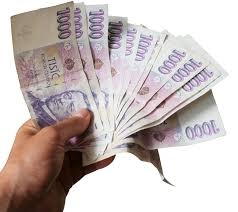Did you find an error or inaccuracy? Feel free to write us. Thank you!Tips to related online calculators
Do you have a system of equations and looking for calculator system of linear equations?

## Related math problems and questions:

• RepairingThree employees earned a total of € 469 for repairing the equipment. They split so that the first got 20% more than the second, and the third 15% more than the second. How many euros did everyone get?
• RewardThree workers shared a common reward 13110 CZK: the first worker got 35% less than the second, and the third worker got 20% more than the second worker. How much got each worker?
• ContestantsThe three best contestants are to divide the total prize of CZK 4,200. The second gets 20% more than the third. And the first one gets 200 CZK less than the second and the third together. How much will everyone get?
• Comparing salariesSpouses compared salaries. Mrs. Janka found that a 20% higher salary than Mr Jaroslav. Together earned € 1,430. How much they earn each of them?
• MerchantThe merchant lower cost by 10% in December when it was not sold. Again in January lower cost by 20% and now costs 576 €. A. How much the goods stood originally? B, how much cost the goods after the first lowering? C, How many percents totally merchant low
• Three workers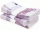The three workers received € 2,850 together for the work done. They divided them according to the time worked so that the first received 20% less than the second and the third € 50 more than the second. How much EUR did each worker receive?
• Part-time workersThree part-time workers received CZK 1,235 for their work. The first received 20% less than the second and the third received 45 CZK more than the second. Determine how many crowns (CZK) each of them received.
• RemunerationTotal remuneration for workers is 47 000 CZK. The second worker received 20% more than the first and third 4000 CZK less than a second employee. How many CZK each received?
• Four numbersThe first number is 50% second, the second number is 40% third, the third number is 20% of the fourth. The sum is 396. What are the numbers?
• Dividing moneyThomas, Honza and Vasek are to divide 1220kč. Honza got 25% more than Thomas. Vasek 20% less than Thomas. How CZK each got?
• The grocery storeThe grocery store earned € 15450 in the first week. In the second € 18630. What percentage did sales increase in the second week compared to the first week?
• Coffee shop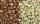To the coffee shop brought 2 types of coffee totally 50 kg. The first type was CZK 220 per kilogram, coffee second type 300 CZK per 1 kg. For all the coffee trader earned CZK 12,000. How many kilograms of coffee of first type and how many kilograms of cof
• ProfitsTwo members will make profit 52 000, - EUR. The forst earned 8% more and the second 10% more and thus earned together 56 700, - EUR. How many will initially each earn?
• Siblings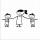Three siblings had saved up a total of 1,274 CZK. Peter had saved up to 15% more than Jirka and Hanka 10% less than Peter. How much money did they save each one of them?
• Sum of three numbersThe sum of three numbers from which second number is 20% smaller than the first number and the third number is 25% smaller than the second number is 96. Determine this numbers.
• Final priceThe book with the original price of CZK 850 was reduced by 20%. In a week, it was raised by 20% of the new price. Find the final price of the book. How much percents of the original price of the book is the final price?
• 3 days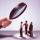Worker checked 2,950 products in 3 days. Second day checked 25% more than the first day. The third day 15% more products than the second day. How many products he checked in each day.